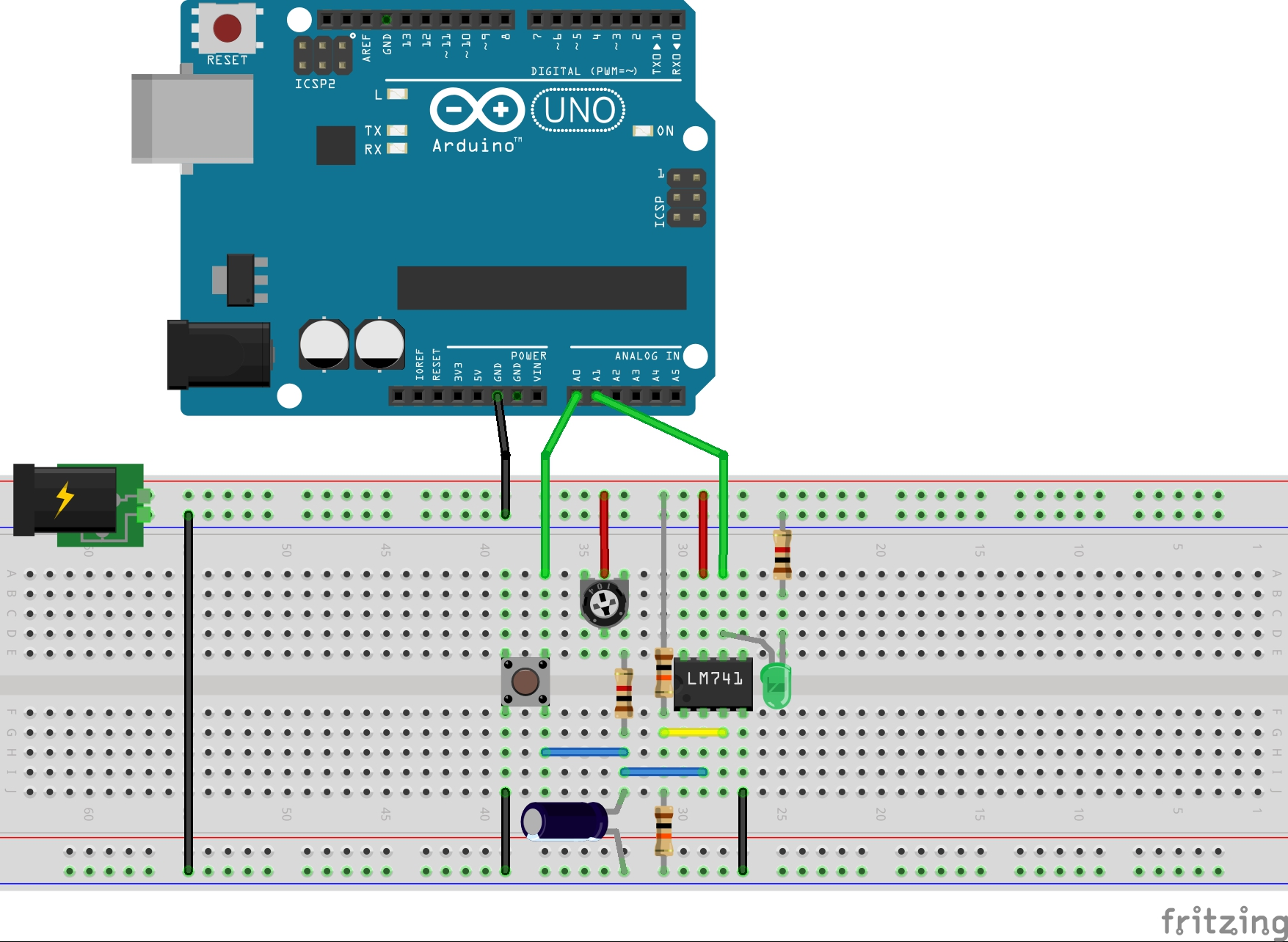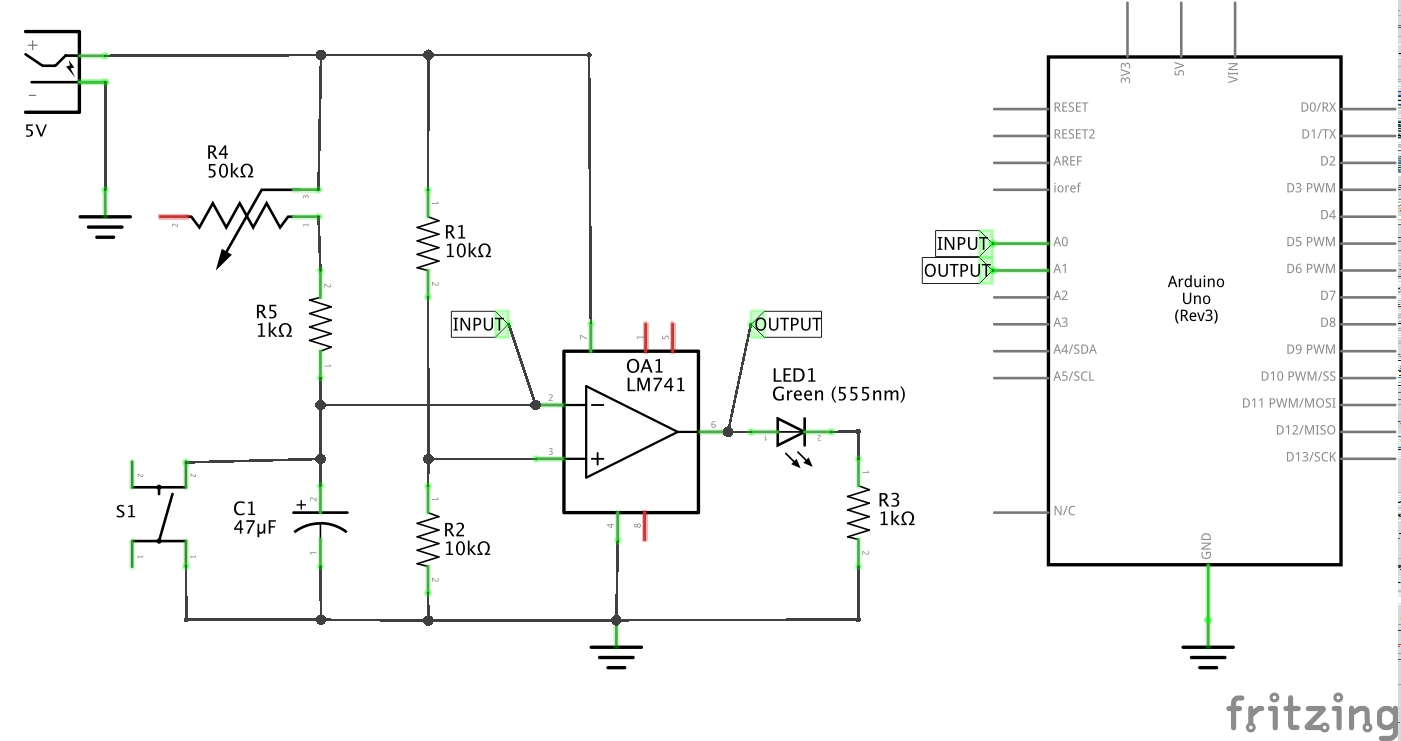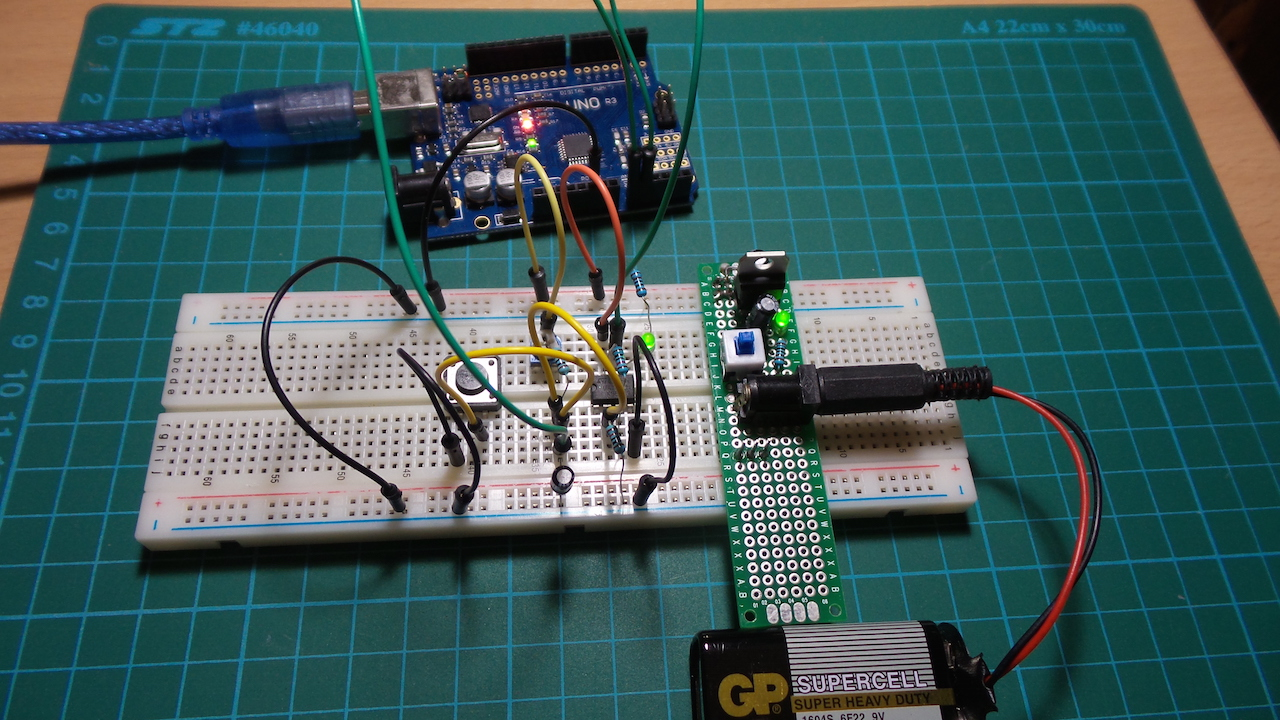# #122 OpAmpTimer

Test an OpAmp timer switch.

This circuit uses an OpAmp configured as a comparator to switch an output for a period of time governed by a variable RC time constant (𝛕).

The comparator switching point is half the supply voltage, set by the R1/R2 voltage divider. So with a 5V supply, the switching point is 2.5V.

At steady-state, C1 is fully charged to positive supply and this sets the inverting OpAmp input well above the non-inverting threshold and therefore the OpAmp output is off (low).

When S1 is pressed, C1 is fully discharged and the inverting OpAmp input pulled low, so OpAmp output swithes on (high).

When S1 is released, C1 charges through R4+R5, and the OpAmp output remains high until the C1 voltage reaches the switching threshold. R4 is a 50kΩ pot, which allows the switching time to be adjusted. R1 is included to set a minimum resistance and avoid a short if R4 is dialed to 0Ω.

The time it takes to switch can be determined from the RC complete response formula. Assuming a 5V supply:

``````v(t) = v(∞) + [v(0) - v(∞)] e^(-t/𝛕)
2.5 = 5 - 5 e^(-t/𝛕)
2.5/5 = e^(-t/𝛕)
ln(0.5) = -t/𝛕
t = - 𝛕 ln(0.5)
``````

So the low and high timing limits are:

• when R4+R5=1kΩ and C=47µF: 32ms
• when R4+R5=51kΩ and C=47µF: 1.6s

Note that the switching logic may be flipped to normally on with a timed off period by switching the inverting/non-inverting inputs.

The LM741 is not capable or rail-to-rail operation. In fact it is not a particularly good OpAmp - but it is convenient. With a 5V supply, the particular unit used here only measures a 2.6V swing:

• output low: 1.6V
• output high: 4.2V

Here’s a sample trace of the circuit behaviour recorded using PlotNValues (a simple Processing sketch)

• upper trace is the OpAmp output
• lower trace is the non-inverting input (i.e. C1 voltage)## Construction

Note: the Arduino is included for measurement purposes only. The circuit does not require it. When a 5V Arduino like the Uno is attached, the circuit should also be powered at 5V. Without the Arduino, the supply could be anything within the operating limits of the OpAmp, with perhaps R3 adjusted to limit current to the LED as required.